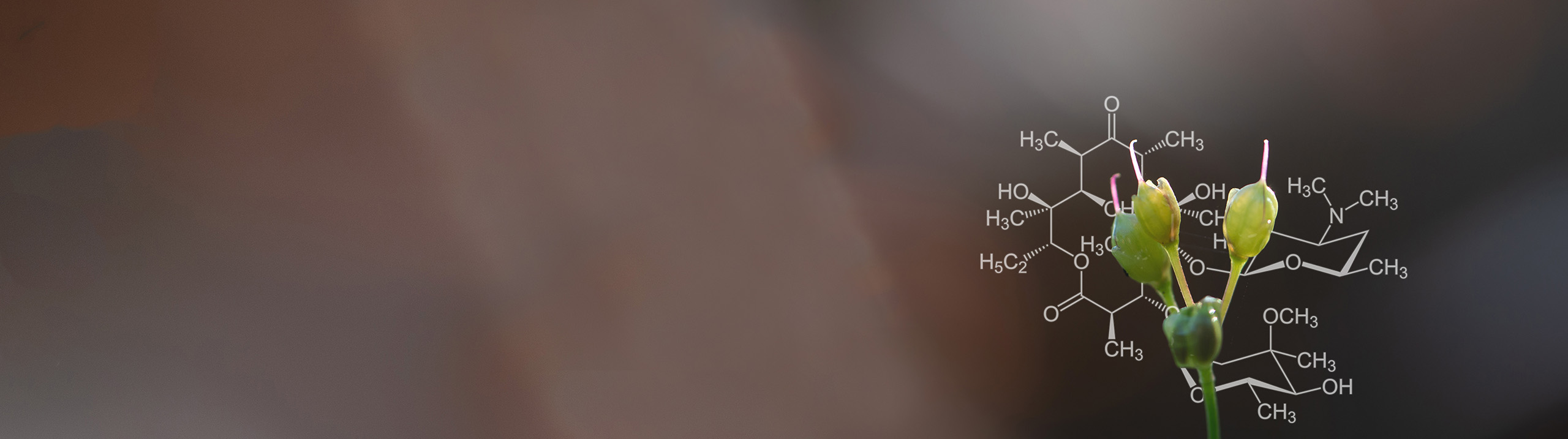Start typing, then use the up and down aroows to select an option from the list.# Biochemistry

Learn the toughest concepts covered in Biochemistry with step-by-step video tutorials and practice problems by world-class tutors

1.Introduction to Biochemistry

# Gibbs Free Energy

1

### concept

Gibbs Free Energy Equation1m
Play a video:
0
2

### concept

Reaction Direction5m
Play a video:
1
3

### concept

Gibbs Free Energy (Standard Conditions)3m
Play a video:
0
4

### concept

Gibbs Free Energy (Physiological Conditions)8m
Play a video:
0
5
Problem

Consider a reaction where Keq=1.6 but Q = 3.19. What direction will the reaction proceed?

6
Problem

At equilibrium, the reaction A ⇌ B + C has the following reactant concentrations: [A] = 3 mM, [B] = 4 mM, and [C] = 10 mM. What is the standard free energy change for the reaction & is it endergonic or exergonic?

7
Problem

ΔG˚=141.7 kJ for the following reaction. Calculate ΔG: T=10˚C, [SO 3] = 25mM, [SO2] = 50mM, & [O2] = 75 mM.

2 SO3(g) ⇌ 2 SO2(g) + O2(g)